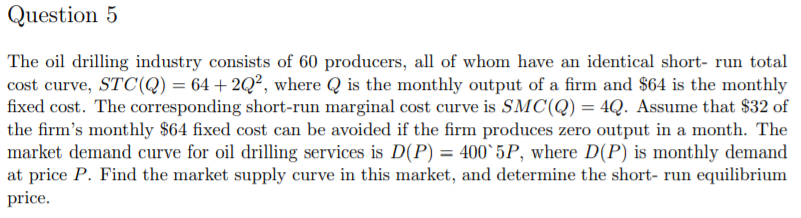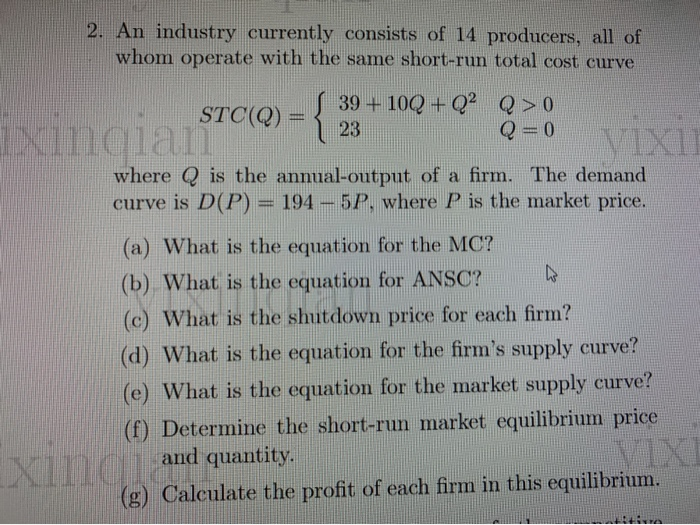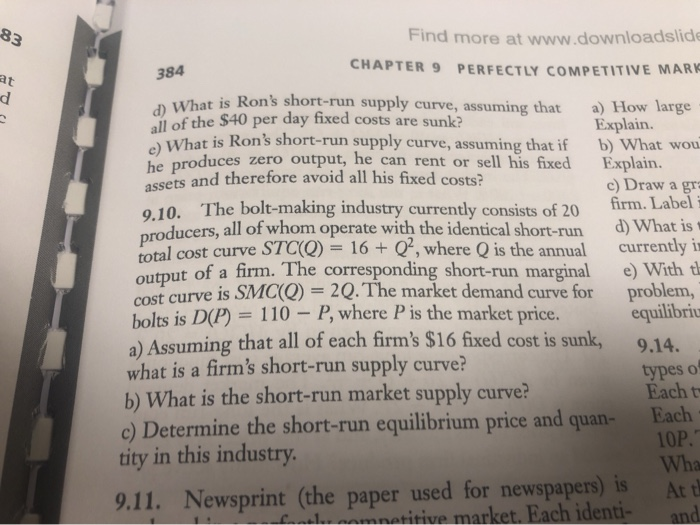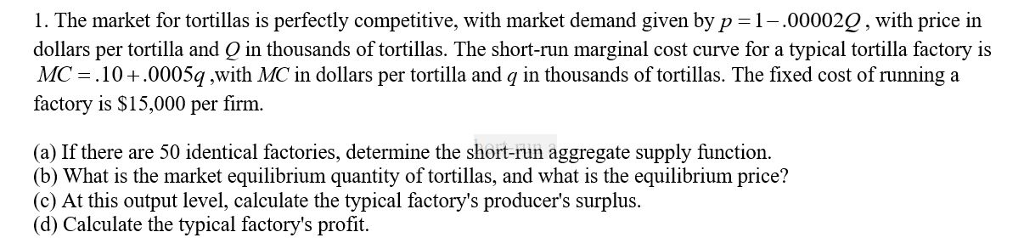# Please answer me in detail. Thank you. Market demand curve is D(P)=400-5P. The oil drilling industry consists of 60 pro...Market demand curve is D(P)=400-5P.

The oil drilling industry consists of 60 producers, all of whom have an identical short- run total cost curve, STC(Q) = 64 + 2Q2, where Q is the monthly output of a firm and \$64 is the monthly fixed cost. The corresponding short-run marginal cost curve is SMC(Q) 4Q. Assume that \$32 of the firm's monthly \$64 fixed cost can be avoided if the firm produces zero output in a month. The market demand curve for oil drilling services is D(P)-400 5P, where D(P) is monthly demand at price P. Find the market supply curve in this market, and determine the short- run equilibrium price

P= SMC= 4Q

Q= P/4

This is the firm's supply function.

Market supply curve= 60*Q= 60*P/4= 15P

At equilibrium, supply= demand

15P= 400-5P

20P= 400

P= 20

##### Add Answer of: Please answer me in detail. Thank you. Market demand curve is D(P)=400-5P. The oil drilling industry consists of 60 pro...
Similar Homework Help Questions
• ### 2. An industry currently consists of 14 producers, all of whom operate with the same short-run...2. An industry currently consists of 14 producers, all of whom operate with the same short-run total cost curve S 39 + 10Q + Q2 STCQ) - STC(Q) = { 23 Q > 0 Q = 0 where Q is the annual-output of a firm. The demand curve is D(P) = 194 – 5P. where P is the market price. (a) What is the equation for the MC? (b) What is the equation for ANSC? (c) What is the shutdown...

• ### Industry X has a market demand curve given by the equation P = 100 – Q/100

Industry X has a market demand curve given by the equation P = 100 – Q/100, where P isthe market price, and Q is industry-wide output.100 perfectly competitive firms currentlyoperate in industry X. Each of these firms has a total cost function given by TC = 100 +10q + q2, where q is the output of the individual firm.What is the market output in the short run? What is the market price? How much doindividual firms produce? Do firms earn...

• ### consider a market with inverse demand curve p=400-4Q. costs per firm are given by C(q)= 16+10q+q^2...

consider a market with inverse demand curve p=400-4Q. costs per firm are given by C(q)= 16+10q+q^2 a) find the minimum efficient scale output level b) in a competitive market , how many firms will be active in the long-run c) suppose we have a cournot oligopoly with n firms . determine output of each firm and the equilibrium price d) find the long run equilibrium number of firms if the market is a cournot oligopoly and entry occurs until profit...

• ### 83 Find more at www.downloadslide CHAPTER 9 PERFECTLY COMPETITIVE MARK 384 D What is Ron's short-run supply curve, assuming that all of the \$40 per day fixed costs are sunk? e) What is Ron&...83 Find more at www.downloadslide CHAPTER 9 PERFECTLY COMPETITIVE MARK 384 D What is Ron's short-run supply curve, assuming that all of the \$40 per day fixed costs are sunk? e) What is Ron's short-run supply curve, assuming that if he produces zero output, he can rent or sell his fixed a) How large Explain. b) What wou Explain. c) Draw a gr firm. Label i and therefore avoid all his fixed costs? The bolt-making industry currently consists of 20...

• ### Consider a market with demand curve ?=200−? and suppose that the industry consists of a dominant...

Consider a market with demand curve ?=200−? and suppose that the industry consists of a dominant firm which has a constant marginal cost equal to \$40 per unit. There are ten other fringe producers; each has a marginal cost curve ??=40+10?, where q is the output of a typical fringe producer. Assume there are no fixed costs for any producer. a. What is the supply curve of the competitive fringe? b. What is the dominant firm’s residual demand curve? c....

• ### 5. Assume a market demand curve of D(P) = 60−2P and a fringe supply curve of S(P) = P − 5. Assume a cost curve for the incumbent of C(Q) = 10 + 4Q. Find the market outcome in terms of price and quanti...

5. Assume a market demand curve of D(P) = 60−2P and a fringe supply curve of S(P) = P − 5. Assume a cost curve for the incumbent of C(Q) = 10 + 4Q. Find the market outcome in terms of price and quantity both for a monopolist not facing a fringe and a large dominant firm facing a fringe. Be sure to both solve for and graph the dominant firm’s demand and marginal revenue curves [in both the regions...

• ### STC = 40 + 10Q + 0.1Q^2 . SMC = 10 + 0.2Q. The market price...

STC = 40 + 10Q + 0.1Q^2 . SMC = 10 + 0.2Q. The market price is \$20. a. Find the profit maximizing Q. b. Calculate the maximum profit. c. Find the average variable cost. d. In the short run, at what price will this firm close? e. Find the firm’s short run supply curve and express it as a function of price: Qs (P) = ?

• ### Microeconomic Theory Question

Problem 9.11, pg 380 of the text Microeconomics:Newsprint (the paper used for newspapers) is produced in a perfectly competitive market. Each identical firm has a total variable cost TVC(Q)= 40Q + 0.5Q2, with anassociated marginal cost curve SMC(Q) = 40 + Q. A firm’s fixed cost isentirely nonsunk and equal to 50.a) Calculate the price below which the firm will not produce any output in the short run.b) Assume that there are 12 identical firms in this industry. Currently, the...

• ### 1. The market for tortillas is perfectly competitive, with market demand given by p 1.000022, with...1. The market for tortillas is perfectly competitive, with market demand given by p 1.000022, with price in dollars per tortilla and Q in thousands of tortillas. The short-run marginal cost curve for a typical tortilla factory is MC 10+.0005q,with MC in dollars per tortilla and q in thousands of tortillas. The fixed cost of running a factory is \$15,000 per firm. (a) If there are 50 identical factories, determine the short-run aggregate supply function. (b) What is the market...

• ### Suppose the market for canola oil is perfectly competitive. There are 1,000 firms in the market,...

Suppose the market for canola oil is perfectly competitive. There are 1,000 firms in the market, each of which have a fixed cost of FC=2 and a marginal cost of MC= 1+Q, where q is quantity produced by an individual firm. Let QS denote the total quantity supplied in the market. The market demand is QD= 15,250-250P A) Find the market supply equation, that is write QS as a function of price P B)What is the equilibrium price? What is...

Need Online Homework Help?